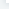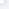# NumPy Matplotlib

NumPy MatplotlibMatplotlib 是 Python 的绘图库。 它可与 NumPy 一起使用，提供了一种有效的 MatLab 开源替代方案。 它也可以和图形工具包一起使用，如 PyQt 和 wxPython。Windows 系统安装 Matplotlib进入到 cmd 窗口下，执行以下命令：python -m pip install -U pip setuptoolspython -m pip install matplotlibLinux 系统安装 Matplotlib可以使用 Linux 包管理器来安装：Debian / Ubuntu：sudo apt-get install python-matplotlibFedora / Redhat：sudo yum install python-matplotlibMac OSX 系统安装 MatplotlibMac OSX 可以使用 pip 命令来安装：sudo python -mpip install matplotlib安装完后，你可以使用 python -m pip list 命令来查看是否安装了 matplotlib 模块。\$ python -m pip list | grep matplotlibmatplotlib (1.3.1)实例实例import numpy as npfrom matplotlib import pyplot as pltx = np.arange(1,11)y = 2 * x + 5plt.title("Matplotlib demo")plt.xlabel("x axis caption")plt.ylabel("y axis caption")plt.plot(x,y) plt.show()以上实例中，np.arange() 函数创建 x 轴上的值。y 轴上的对应值存储在另一个数组对象 y 中。 这些值使用 matplotlib 软件包的 pyplot 子模块的 plot() 函数绘制。图形由 show() 函数显示。图形中文显示Matplotlib 默认情况不支持中文，我们可以使用以下简单的方法来解决：首先下载字体（注意系统）：https:

//www.fontpalace.com/font-details/SimHei/SimHei.ttf 文件放在当前执行的代码文件中：实例import numpy as npfrom matplotlib import pyplot as pltimport matplotlib

# fname 为 你下载的字体库路径，注意 SimHei.ttf 字体的路径zhfont1 = matplotlib.font_manager.FontProperties(fname="SimHei.ttf")x = np.arange(1,11)y = 2 * x + 5plt.title("k88.net - 测试", fontproperties=zhfont1)

# fontproperties 设置中文显示，fontsize 设置字体大小plt.xlabel("x 轴", fontproperties=zhfont1)plt.ylabel("y 轴", fontproperties=zhfont1)plt.plot(x,y)plt.show()执行输出结果如下图：此外，我们还可以使用系统的字体：from matplotlib import pyplot as pltimport matplotliba=sorted([f.name for f in matplotlib.font_manager.fontManager.ttflist])for i in a:

print(i)打印出你的 font_manager 的 ttflist 中所有注册的名字，找一个看中文字体例如：STFangsong(仿宋）,然后添加以下代码即可：plt.rcParams['font.family']=['STFangsong']作为线性图的替代，可以通过向 plot() 函数添加格式字符串来显示离散值。 可以使用以下格式化字符。字符描述'-'实线样式'--'短横线样式'-.'点划线样式':

'虚线样式'.'点标记','像素标记'o'圆标记'v'倒三角标记'^'正三角标记'<'左三角标记'>'右三角标记'1'下箭头标记'2'上箭头标记'3'左箭头标记'4'右箭头标记's'正方形标记'p'五边形标记'*'星形标记'h'六边形标记 1'H'六边形标记 2'+'加号标记'x'X 标记'D'菱形标记'd'窄菱形标记'&

#124;'竖直线标记'_'水平线标记以下是颜色的缩写：字符颜色'b'蓝色'g'绿色'r'红色'c'青色'm'品红色'y'黄色'k'黑色'w'白色要显示圆来代表点，而不是上面示例中的线，请使用 ob 作为 plot() 函数中的格式字符串。实例import numpy as npfrom matplotlib import pyplot as pltx = np.arange(1,11)y = 2 * x + 5plt.title("Matplotlib demo")plt.xlabel("x axis caption")plt.ylabel("y axis caption")plt.plot(x,y,"ob")plt.show()执行输出结果如下图：绘制正弦波以下实例使用 matplotlib 生成正弦波图。实例import numpy as npimport matplotlib.pyplot as plt

# 计算正弦曲线上点的 x 和 y 坐标x = np.arange(0, 3 * np.pi, 0.1)y = np.sin(x)plt.title("sine wave form")

# 使用 matplotlib 来绘制点plt.plot(x, y)plt.show()执行输出结果如下图：subplot()subplot() 函数允许你在同一图中绘制不同的东西。以下实例绘制正弦和余弦值:

# 计算正弦和余弦曲线上的点的 x 和 y 坐标 x = np.arange(0, 3 * np.pi, 0.1)y_sin = np.sin(x)y_cos = np.cos(x)

# 建立 subplot 网格，高为 2，宽为 1

# 激活第一个 subplotplt.subplot(2, 1, 1)

# 绘制第一个图像 plt.plot(x, y_sin)plt.title('Sine')

# 将第二个 subplot 激活，并绘制第二个图像plt.subplot(2, 1, 2)plt.plot(x, y_cos)plt.title('Cosine')

# 展示图像plt.show()执行输出结果如下图：bar()pyplot 子模块提供 bar() 函数来生成条形图。 以下实例生成两组 x 和 y 数组的条形图。实例from matplotlib import pyplot as pltx = [5,8,10]y = [12,16,6]x2 = [6,9,11]y2 = [6,15,7]plt.bar(x, y, align = 'center')plt.bar(x2, y2, color = 'g', align = 'center')plt.title('Bar graph')plt.ylabel('Y axis')plt.xlabel('X axis')plt.show()执行输出结果如下图：numpy.histogram()numpy.histogram() 函数是数据的频率分布的图形表示。 水平尺寸相等的矩形对应于类间隔，称为 bin，变量 height 对应于频率。numpy.histogram()函数将输入数组和 bin 作为两个参数。 bin 数组中的连续元素用作每个 bin 的边界。实例import numpy as npa = np.array([22,87,5,43,56,73,55,54,11,20,51,5,79,31,27])np.histogram(a,bins = [0,20,40,60,80,100])hist,bins = np.histogram(a,bins = [0,20,40,60,80,100])print (hist)print (bins)输出结果为：[3 4 5 2 1][ 0 20 40 60 80 100]plt()Matplotlib 可以将直方图的数字表示转换为图形。 pyplot 子模块的 plt() 函数将包含数据和 bin 数组的数组作为参数，并转换为直方图。实例from matplotlib import pyplot as pltimport numpy as npa = np.array([22,87,5,43,56,73,55,54,11,20,51,5,79,31,27])plt.hist(a, bins = [0,20,40,60,80,100])plt.title("histogram")plt.show()执行输出结果如下图：Matplotlib 更多参考内容：Matplotlib 教程用户指南常见问题及回答截屏

NumPy Matplotlib

## 相关文章